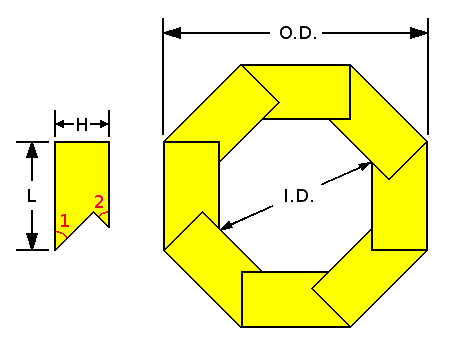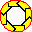Bird's Mouth Spar Size Calculators
 By Gaétan Jett� - Sherbrooke, CanadaIntroduction

Some years ago, a rather long article on birdsmouth's spars (written by me) was published on this Web site. Buried deep down in that article were calculators for birdsmouth's spars size calculation. Some people have expressed the desire to have a quicker access to these calculators. So here they are, on a separate page. This should work much faster if you have an old computer.

I've added two bonus features: first, two new calculators for modified bird's mouth spars. Second there's also a downloadable version at the bottom of the page. That way, once installed, you could use it on your computer even if your Internet connection is down. Enjoy.

Gaétan Jetté

Instructions

The Calculators will work with most browsers out there (Internet Explorer, Firefox, Google Chrome, Opera, Netscape). Internet Explorer users may get a message that some scripts were blocked for security reasons when they open the page. If so, you will have to click on that message to authorize the scripts, otherwise the calculators won't work. These Calculators are written in a language called Java Script. Java Script is a limited programming language that cannot touch your files. It is safe to authorize these scripts. All the other browsers mentioned will work without any hassle. (Take that, Microsoft!)

Choose the Calculator that suits your needs. Enter the values of your choice on the left, then click on the "Calculate!" button. Results will appear on the right. An error message will appear in the Status box if your inputs don't work out. You can clear all entries by clicking on the Reload or Refresh button of your Web browser. Or you can just enter new inputs and click on "Calculate!" again. Clicking on the pictogram in the middle will lead you to Figure 1, in case you need a reminder for all those definitions. Dimensions must all be in the same units. Ex.: Inputs in inches give results in inches.

Please note that the value of O.D. is for a rounded exterior. That detail is especially important if you ever consider doing a modified birdsmouth spar with an odd number of sides.

Hints to avoid error messages (in the Status box)

Input values must be numbers higher than zero (no negatives, no letters).
The number of sides must be 5 or more.
I.D. must be smaller than O.D. (obviously).
H or K must not be too small or too large (less obvious).
H must be smaller than the radius or half the diameter.

Calculator 1: Given N, calculate the two angles
used for the V-notch on the staves.
 Input N: (Number of sides)
 Results Angle 1: degrees Angle 2: degrees

Status :

Calculator 2: Given N, O.D. and I.D.,
calculate L, H and K
 Inputs N: O.D.: I.D.:
 Results L : H : A : K : M :

Status :

Calculator 3: Given N, L and H,
calculate O.D., I.D. and K
 Inputs N : L : H :
 Results O.D.: I.D.: A : K : M :

Status :

Calculator 4: Given N, O.D. and H,
calculate L, I.D. and K
 Inputs N : O.D.: H :
 Results L : I.D.: A : K : M :

Status :

Calculator 5: Given N, O.D. and K,
calculate L, H and I.D.
 Inputs N : O.D.: K :
 Results L : H : I.D.: A : M :

Status :

Calculator 6: Given N, L and K,
calculate O.D., H and I.D.
 Inputs N : L : K :
 Results O.D.: H : I.D.: A : M :

Status :Figure 1- Bird's Mouth Spar : Definitions

 O.D. is the outside diameter I.D. is the largest inside diameter R1 is the shortest inner radius R2 is the largest inner radius or half I.D. N is the number of sides (eight in this case) a (alpha) is the angle between adjacent staves or 360° / N L is the width of material H is the thickness of material Important Ratios K is the thickness to width ratio of staves or (H / L) M is the "conversion factor" or (O.D. / L) A is the inside to outside diameter ratio or (I.D. / O.D.)

Bonus Feature! Modified Birdsmouth Spar Calculators

Modified bird's mouth spars have their staves cut differently so that their outside edges fit flush together, creating a clean polygon. The size formula is not the same as the "regular" or traditional birdsmouth. Hence these new calculators.

Calculator 7: Given N, O.D. and I.D.,
calculate L, H and K
 Inputs N: O.D.: I.D.:
 Results L : H : A : K : M :

Status :

Calculator 8: Given N, L and H,
calculate O.D., I.D. and K
 Inputs N : L : H :
 Results O.D.: I.D.: A : K : M :

Status :Figure 2- Modified Bird's Mouth Spar : Definitions

 O.D. is the outside diameter I.D. is the largest inside diameter 1 is the first angle of the stave 2 is the second angle of the stave L is the width of material measured on the outside H is the thickness of materialDownload bird-calc.zip Size: 33.7 KBsails plans epoxy rope/line hardware canoe/Kayak sailmaking materials models media tools gear